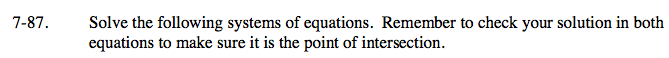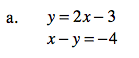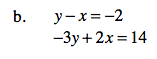### Home > CAAC > Chapter 7 > Lesson 7.3.1 > Problem7-87

7-87.One way to solve this system of equations is by using the substitution method.

y = 2x − 3. Substitute y:
xy = −4
x − (2x−3) = −4

Solve for x.

Distribute the left side.

x − 2x + 3 = −4

Combine like terms.

x + 3 = −4

Subtract 3 on both sides.

x = −7

Use the solution you found for x and substitute it into either original equation and solve for y.

x = 7

y = 11One way to solve this system of equations is by using the elimination method.

Multiply the top equation by 2.

2(yx = − 2)
2y − 2x = −2

Add the two equations together by combining like terms.

2y − 2x = −4
−3y + 2x = 14
y + 0 = 10

Solve for y.

When you find y, you can now subsitute the value into either original equation and solve for x.

Don't forget to check your solution!